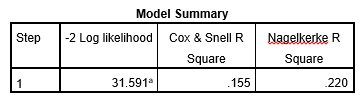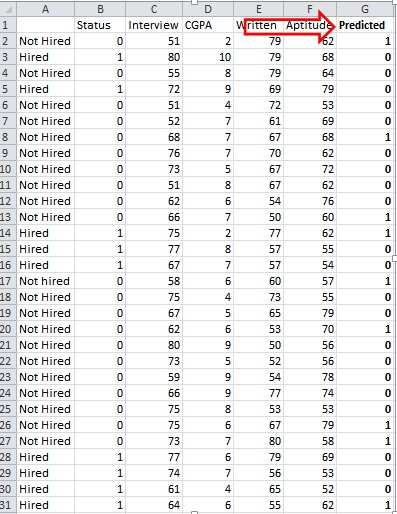# How to apply logistic regression in a case?

Machine learning involves solutions to predict scenarios based on past data. Logistic regression offers probability functions based on inputs and their corresponding output. In short, the dependent variable is a classification variable.

One of the most common methods of data analysis is the linear or multiple regression analysis. It helps establish the relationship between independent and dependent variables. In linear regression the dependent variable is continuous in most cases. However,  if the dependent variable is categorical then simple regression will not allow for prediction. So, statisticians introduced the logistic regression method where the dependent variable is non-continuous or it is categorical. Like all regression, it is also used in predictive analysis. However, it helps describe the behavior of a variable which is binary, i.e., which can take only two values. If there are more than two categories in the dependent variable, then multinomial logistic regression is applicable instead of simple logistic regression. Below is an example of how this test works.

## Application of logistic regression

This section contains a case study to explain the application of logistic regression on a dataset. The case dataset here contains two series, series X and Y. Series X indicates the CGPA (Cumulative Grade Points Average) of ten students of a school. Series Y indicates whether these students gets admission in college, where 1= admit, and 0=no admit. The table below represents their values.

 (CGPA) X (Admission)Y 6 0 4 0 7.7 1 10 1 8.9 1 5.5 0 6.7 0 8.9 1 7.6 1 8.7 1

Table 1: Values for logistic regression case 1

Here, logistic regression will help assess what level of CGPA leads to admission in college. This is called a ‘binary classification’ (either 1 or 0) problem. These types of cases need logistic regression. The aim here is to come up with the probability function that takes input X (CGPA) with outcome ‘what is the probability of getting admission’. Hence this test estimates a probability of each student based on its score. Furthermore, based on that probability, the analysis predicts if the student will get admission or not.

Logistic regression analysis is used to get the outcome for following expressions:This function is also called the ‘log of odds’ of an event. On the basis of the above log of odds function the multiple regression equation for logistic regression can be:

##Logistic regression case

Undertaken again a case study of students appearing for job interview, the aim is to determine the applicability of scores of interviews, CGPA, written and aptitude tests in hiring.Image 1: Logistic regression case data

The above data was processed using SPSS. The images below show the results of the test on the above data. The first table displays the model summary and the significance level. They indicate if the undertaken variables were enough to ascertain the job status.a. Estimation terminated at iteration number 5 because parameter estimates changed by less than .001. Image 2: Model summary from the logistic regression.

Here, Cox & Snell R squared and Nagelkerke R squared are similar to the R squared in the normal linear regression analysis. This explains the variation in the dependent variable due to independent variables included in the logistic model.Image 3: Results of the test

The second table list outs the SPSS generated ‘predicted’ job status, based on the trends in undertaken variables.Image 4: Predicted value from the logistic regression analysis

## Applications of logistic regression

When classification is needed on the dependent variable, this test is applicable. Below are some examples showing its application.

• A credit card company can apply logistic regression to categorize the people in two types; good credit and bad credit based on the characteristics such as annual salary, monthly credit card payments and number of defaults.
• A hospital can apply this test to classify patients into critical and non-critical categories based on selected health attributes.
• Insurance companies use logistic regression to predict the chances that the policy holder will die before the term of policy expires based on characteristics like gender, age and physical examination.
• A bank may use this test to predict the chances that a loan applicant will default on loan or not, based on past debts, past defaults and annual income.
• Marketers may use it in examining whether a customer will respond to a particular ad based on preference of customer and type of web portal customer is active.

## Software supporting logistic regression

Software that support this test with multiple independent variables are R, SAS, MATLAB, STATA and SPSS.

### Priya Chetty

Partner at Project Guru
Priya is a master in business administration with majors in marketing and finance. She is fluent with data modelling, time series analysis, various regression models, forecasting and interpretation of the data. She has assisted data scientists, corporates, scholars in the field of finance, banking, economics and marketing.

### Related articles

• How to apply linear discriminant analysis? Linear discriminant model is a multivariate model. It is used for modeling the differences in groups. In this model a categorical variable can be predicted through continuous or binary dependent variable.
• How to conduct generalized least squares test? In statistics, Generalized Least Squares (GLS) is one of the most popular methods for estimating unknown coefficients of a linear regression model when the independent variable is correlating with the residuals.
• How to perform nonlinear regression? Regression analysis is a statistical tool to study the relationship between variables. These variables are the outcome variable and one or more exposure variables. In other words, regression analysis is an equation which predicts a response from the value of a certain predictor.
• How to conduct path analysis? Path analysis is a graphical representation of multiple regression models. In this analysis, the graphs represent the relationship between dependent and independent variables with the help of square and arrows.
• Understanding random operating curves or ROC analysis Receiver Operating Curve (ROC) is an extension of such classifications. Performance of binary classifier system in the case of ROC analysis can be tested.We are looking for candidates who have completed their master's degree or Ph.D. Click here to know more about our vacancies.